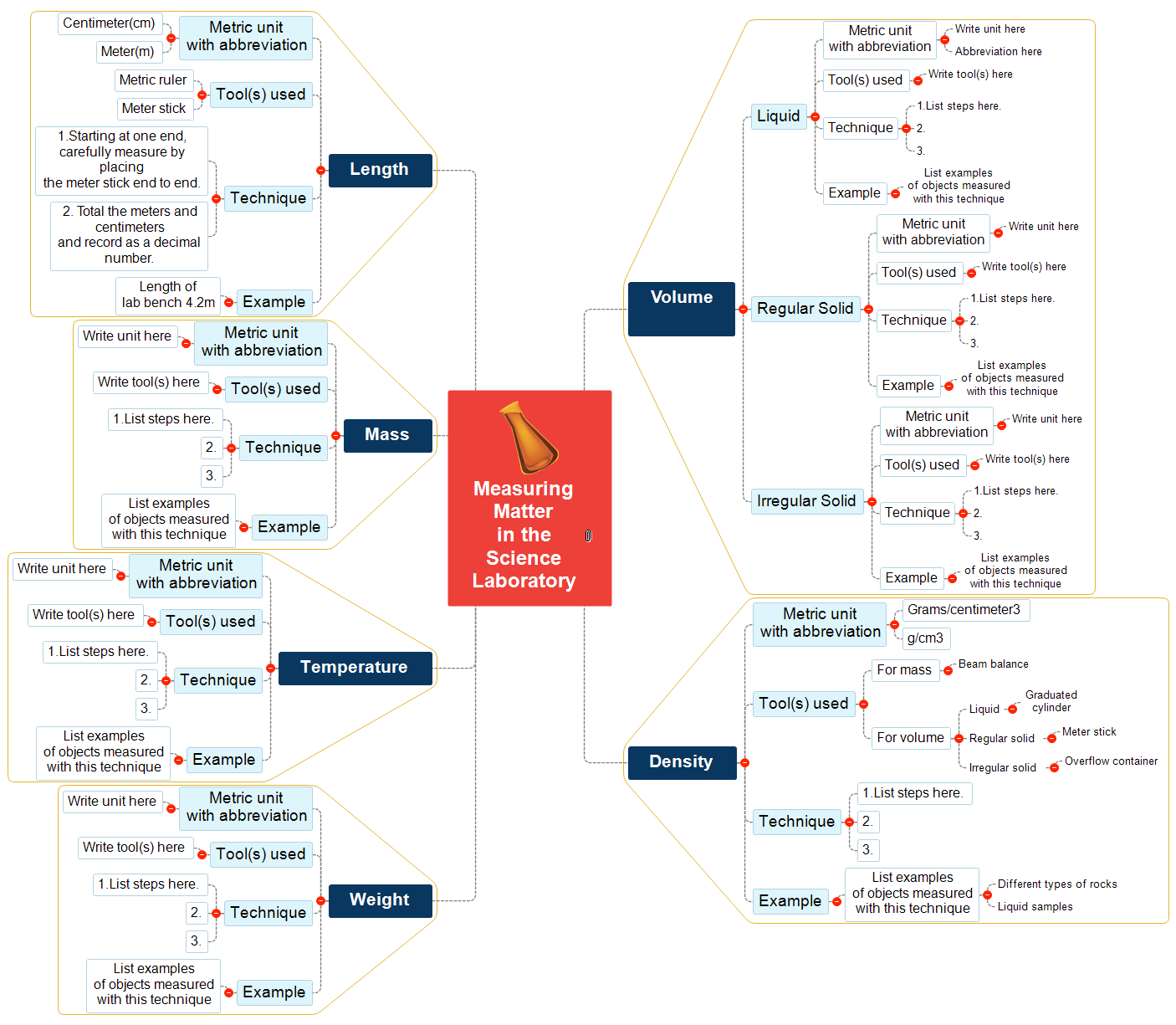Start Mind Mapping Now!
• Professional Mind Mapping
• Best MS Office Integration
• Build-in Gantt chart & Timeline
• Templates & Tutorials

# Measuring Matter - Mind Map## Measuring Matter - Mind Map

4 years ago by: MatchWare

• Measuring Matter in the Science Laboratory
• Volume
• Liquid
• Metric unit with abbreviation
• Write unit here
• Abbreviation here
• Tool(s) used
• Write tool(s) here
• Technique
• 1.List steps here.
• 2.
• 3.
• Example
• List examples of objects measured with this technique
• Regular Solid
• Metric unit with abbreviation
• Write unit here
• Tool(s) used
• Write tool(s) here
• Technique
• 1.List steps here.
• 2.
• 3.
• Example
• List examples of objects measured with this technique
• Irregular Solid
• Metric unit with abbreviation
• Write unit here
• Tool(s) used
• Write tool(s) here
• Technique
• 1.List steps here.
• 2.
• 3.
• Example
• List examples of objects measured with this technique
• Density
• Metric unit with abbreviation
• Grams/centimeter3
• g/cm3
• Tool(s) used
• For mass
• Beam balance
• For volume
• Liquid
• Graduated cylinder
• Regular solid
• Meter stick
• Irregular solid
• Overflow container
• Technique
• 1.List steps here.
• 2.
• 3.
• Example
• List examples of objects measured with this technique
• Different types of rocks
• Liquid samples
• Weight
• Metric unit with abbreviation
• Write unit here
• Tool(s) used
• Write tool(s) here
• Technique
• 1.List steps here.
• 2.
• 3.
• Example
• List examples of objects measured with this technique
• Temperature
• Metric unit with abbreviation
• Write unit here
• Tool(s) used
• Write tool(s) here
• Technique
• 1.List steps here.
• 2.
• 3.
• Example
• List examples of objects measured with this technique
• Mass
• Metric unit with abbreviation
• Write unit here
• Tool(s) used
• Write tool(s) here
• Technique
• 1.List steps here.
• 2.
• 3.
• Example
• List examples of objects measured with this technique
• Length
• Metric unit with abbreviation
• Centimeter(cm)
• Meter(m)
• Tool(s) used
• Metric ruler
• Meter stick
• Technique
• 1.Starting at one end, carefully measure by placing the meter stick end to end.
• 2. Total the meters and centimeters and record as a decimal number.
• Example
• Length of lab bench 4.2m
More Maps by This User